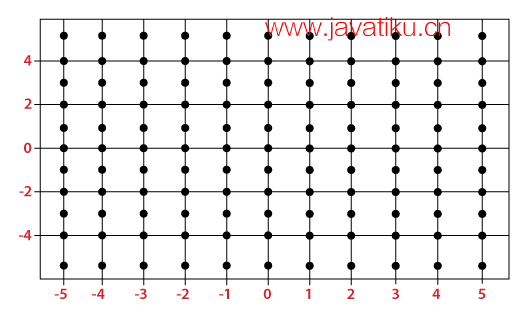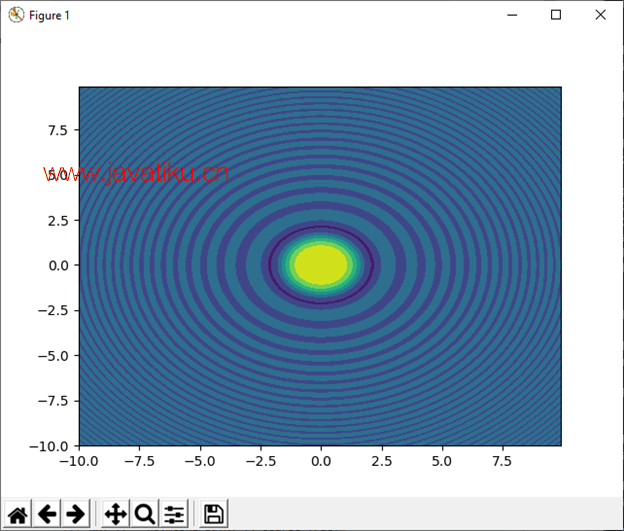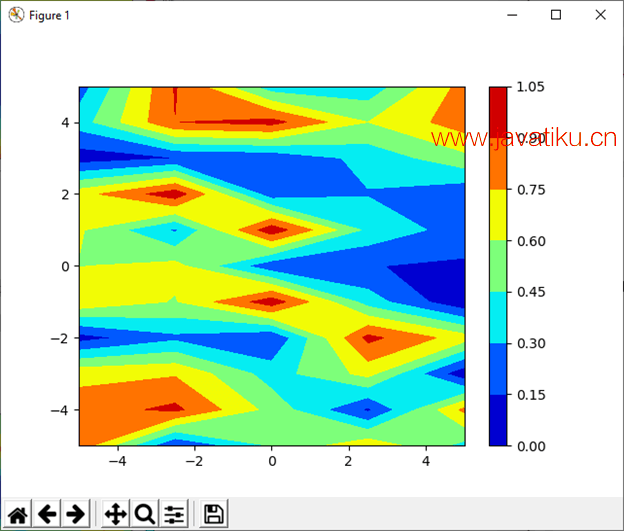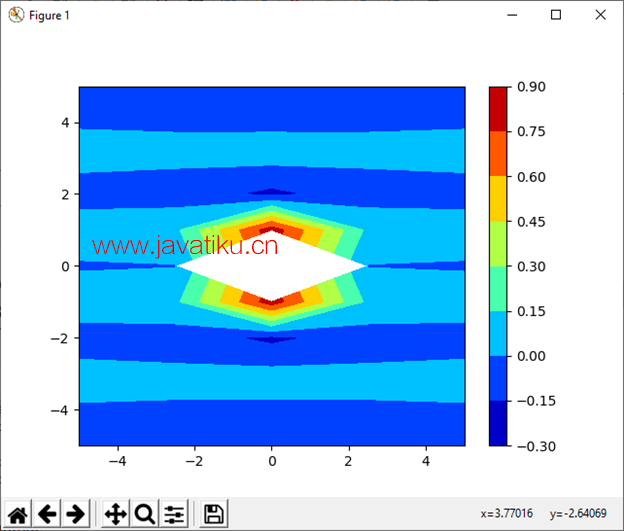# NumPy教程-numpy.meshgrid()在Python中的使用Python的numpy模块提供了meshgrid()函数，用于根据给定的表示矩阵索引或笛卡尔索引的一维数组创建一个矩形网格。MATLAB在一定程度上启发了meshgrid()函数。通过坐标向量，meshgrid()函数返回坐标矩阵。### 语法

``numpy.meshgrid(*xi, **kwargs)``

### 参数

x1, x2,…, xn : array_like

indexing: {'xy', 'ij'}（可选）

sparse: bool（可选）

copy: bool（可选）

X1, X2, …, Xn

### 示例 1：

``````import numpy as np
na, nb = (5, 3)
a = np.linspace(1, 2, na)
b = np.linspace(1, 2, nb)
xa, xb = np.meshgrid(a, b)
xa
xb  ``````

``````array([[1.  , 1.25, 1.5 , 1.75, 2.  ],
[1.  , 1.25, 1.5 , 1.75, 2.  ],
[1.  , 1.25, 1.5 , 1.75, 2.  ]])
array([[1. , 1. , 1. , 1. , 1. ],
[1.5, 1.5, 1.5, 1.5, 1.5],
[2. , 2. , 2. , 2. , 2. ]])``````

• 我们使用别名np导入了numpy模块。
• 我们创建了两个变量，na和nb，并分别赋值为5和3。
• 我们使用linspace()函数创建了两个数组，a和b。
• 然后，我们声明了变量'xa'和'xb'，并将meshgrid()的返回值赋给它们。
• 我们在函数中传递了数组'a'和'b'。
• 最后，我们尝试打印变量'xa''xb'

### 示例 2：

``````import numpy as np
na, nb = (5, 3)
a = np.linspace(1, 2, na)
b = np.linspace(1, 2, nb)
xa, xb = np.meshgrid(a, b, sparse=True)
xa
xb  ``````

``````array([[1.  , 1.25, 1.5 , 1.75, 2.  ]])
array([[1. ],
[1.5],
[2. ]])``````

### 示例 3：

``````import numpy as np
import matplotlib.pyplot as plt
a = np.arange(-10, 10, 0.1)
b = np.arange(-10, 10, 0.1)
xa, xb = np.meshgrid(a, b, sparse=True)
z = np.sin(xa**2 + xb**2) / (xa**2 + xb**2)
h = plt.contourf(a,b,z)
plt.show()  ``````### 示例 4：

``````import numpy as np
import matplotlib.pyplot as plt
a = np.linspace(-5, 5, 5)
b = np.linspace(-5, 5, 11)
random_data = np.random.random((11, 5))
xa, xb = np.meshgrid(a, b)
plt.contourf(xa, xb, random_data, cmap = 'jet')
plt.colorbar()
plt.show()  ``````### 示例 5：

``````import numpy as np
import matplotlib.pyplot as plt
a = np.linspace(-5, 5, 5)
b = np.linspace(-5, 5, 11)
random_data = np.random.random((11, 5))
xa, xb = np.meshgrid(a, b)
sine = (np.sin(xa**2 + xb**2))/(xa**2 + xb**2)
plt.contourf(xa, xb, sine, cmap = 'jet')
plt.colorbar()
plt.show()  ``````